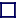### SCIENTIFIC CONSTANTS

Speed of Light (in vacuum), c
Is exactly 2.99792458 × 1010 cm/sec (by definition)

Universal constant of Gravitation, G
G is approximately 66.7259×10-12 m3kg-1s-2.

atomic mass unit, amu (sometimes called a 'Dalton')
The mass of carbon-12 is exactly 12 amu, by definition, making one amu=1.6605402 × 10-27kg.

mass of an electron, me
5.485798959 × 10-4 amu

mass of proton, mp
1.007276487 amu

mass of neutron, mn
1.00866491574 amu

mass of Hydrogen-1, mn
1.00782503207 amu

mass of Helium-4, mn
4.00260325415 amu

mass of muon, mµ
1.8835327 × 10-30 kg

Conversion Factor for mass to energy, amu to MeV
1 amu = 931.494009 MeV

electron Volt, eV
A measure of energy. One electon volt is the amount of energy an electron receives when it is accelaerated by an eletric field with a one volt potential difference.
1eV=160.217733 × 10-21 joules.

Charge on the electron = 160.217733 × 10-21 coulombs

Magnetic moment of electron = 2.00231930436170

Plancks Constant = 662.60755 × 10-36 joule seconds.

Fermi
A unit of distance used in the measurement of nuclear distances. 1 Fermi=10-15metres.

Angstrom, A
A very small unit used to measure atomic distance. 1Angstrom= 1.00001481 × 10-10metres.

Light Year, Astronomical Unit and Parsec
A measure of distance, equal to the distance that light travels in one tropical year. This is equal to 9.46052973 × 1015 metres, which is equal to 6.32388 AU (astronomical units) or 0.306591 parsecs.

Hubbles Constant, Ho
A measure of how fast the Universe is expanding. The reciprocal of the Hubble Constant gives the age of the Universe.
The most accurate value determined so far was in 2003 by using WMAP date, and it comes out at Ho=71 ±5% km/s/Mpc, which means that at a distance of 1 MegaParsec, the Universe is expanding at 71km/s, and at 2MPc 142km/s, etc. A Hubble Constant of 71km/s/MPc would imply a Universe 13.81×109 years old.

The fine structure constant, alpha, is currently known to great precision, being equal to 1/137.035999710.